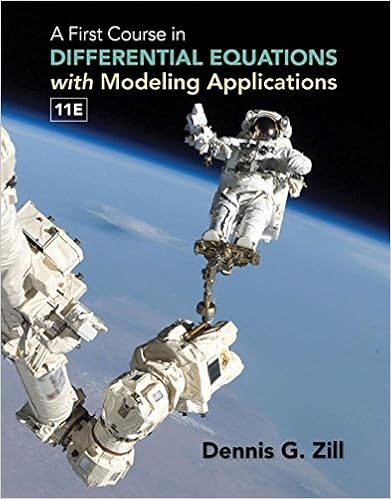# MATH-2070U-Midterm-2010-B-Solutions - MATH 2070U Midterm...

• Test Prep
• Jackjack1000
• 6
• 100% (2) 2 out of 2 people found this document helpful

This preview shows page 1 - 3 out of 6 pages.

##### We have textbook solutions for you!
The document you are viewing contains questions related to this textbook.The document you are viewing contains questions related to this textbook.
Chapter 9 / Exercise 8
A First Course in Differential Equations with Modeling Applications
ZillExpert Verified
MATH 2070UMidterm examinationPage 3 of 81.(a) Assume the variablesA,B,C, andbhave been defined in aMatlabsession as follows.A = [ -1, 3 ;5,4 ];B = [6, 1 ; -2, -4 ];C = [5, -2 ; -3, -4 ;7, -3 ];D = [ -5, -3, -1 ; -1,4,5 ];With these values, what results do the followingMatlabcommands produce.If the statementfails to evaluate, writeERRORwith a short comment explaining why.i. [3 marks]-3 * B .* A .^2i.ii. [3 marks]
##### We have textbook solutions for you!
The document you are viewing contains questions related to this textbook.The document you are viewing contains questions related to this textbook.
Chapter 9 / Exercise 8
A First Course in Differential Equations with Modeling Applications
ZillExpert Verified
MATH 2070UMidterm examinationPage 4 of 8(b) [11/2marks] Write a few lines ofMatlabcode to illustrate how you would determineallnumericalsolutions of the equation6x3= 15x-4.(?)using built-inMatlabroutines. You may assume that the equation (?) has solutions nearx= 0,x= 1, andx= 2.(b)(c) [11/2marks] What is the output of the followingMatlabcommands?
•••